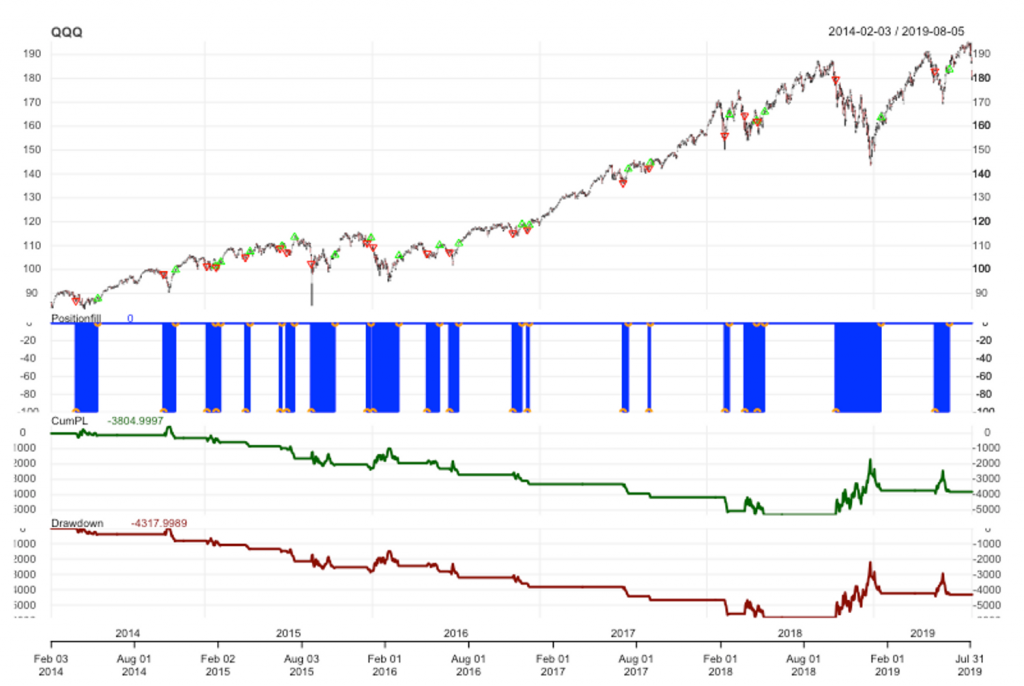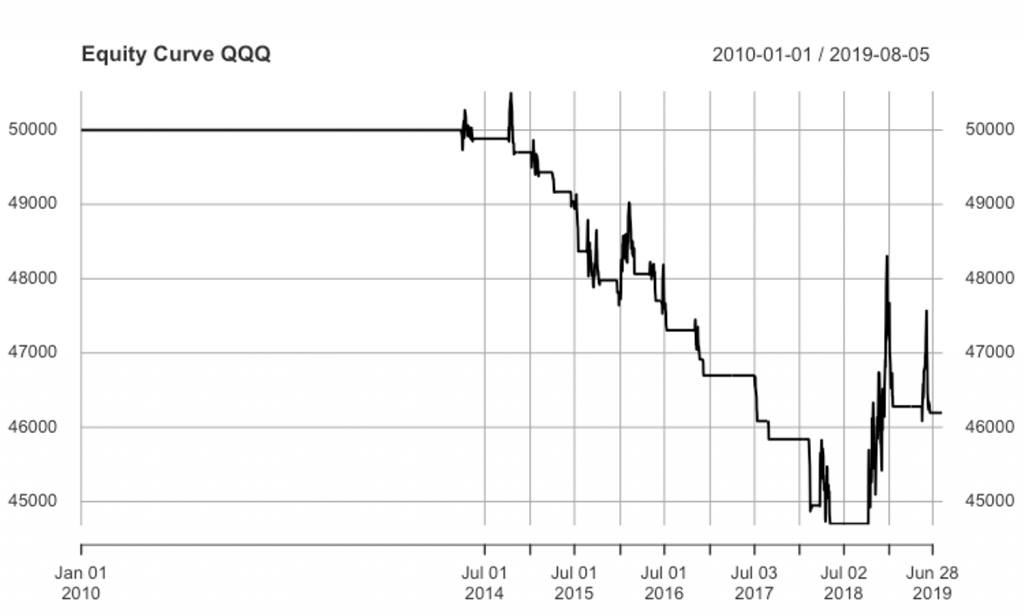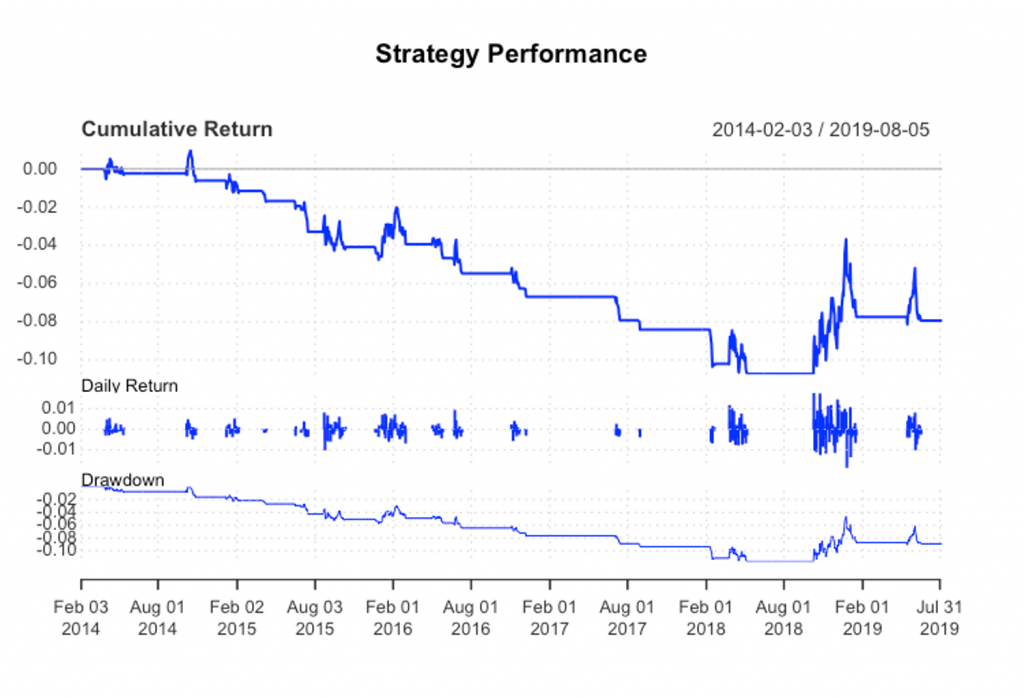# Quantstrat - EMA Crossover Strategy - Performance and Risk Metrics

Once we have the strategy results, quantstrat provides many functions to analyze the strategy and observe important metrics of performance and risk. We would combine the quantstrat package with the PerformanceAnalytics package to show important performance and risk metrics in a trading strategy. We will look at the following:

• Plot the Strategy Performance
• Strategy Statistics and Stats per Trade
• Portfolio Returns
• Account Summary and Equity Curve
• Portfolio Summary and Strategy Performance
• Equity Return Distribution

### Plot the Strategy Performance

We can plot the performance using the chart.Posn(). The chart.Posn() takes two parameters that are portfolio name and the symbol string and return a chart with the symbol price series, the accumulated profit loss and the Drawdown charts. The trades of the strategy are marked in green and red on the price series chart.

# Chart Performance of the Strategy

chart.Posn(portfolioName, Symbol = symbolstring)



#### QQQ EMA Crossover Prices, Strategy P&L and Drawdowns### Strategy Statistics

tradeStats() function calculates statistics about the strategy. These statistics are related to measures about the strategy profits, risk metrics and general features of the strategy such as number of transactions, highest profitable trade, highest loser trade, average profit per trade among others.  In our example, we have the following information:

tstats <- tradeStats(portfolioName)

tstats <- data.frame(t(tstats))

colnames(tstats)

tstats

QQQ
Portfolio          FirstPortfolio
Symbol                        QQQ
Num.Txns                       42
Largest.Winner               1590
Largest.Loser           -882.0001
Gross.Profits            2127.999
Gross.Losses            -5722.998
Percent.Positive         14.28571
Percent.Negative         85.71429
Profit.Factor           0.3718328
Avg.Daily.PL            -171.1905
Med.Daily.PL            -231.9994
Std.Dev.Daily.PL         485.9721
Std.Err.Daily.PL         106.0478
Ann.Sharpe              -5.592018
Max.Drawdown            -5822.998
Profit.To.Max.Draw     -0.6534434
Avg.WinLoss.Ratio        2.230997
Med.WinLoss.Ratio        1.388092
Max.Equity               512.9992
Min.Equity              -5309.999
End.Equity                  -380


The perTradeStats() function takes two arguments that are the portfolio name and the symbols strings and outputs the statistics and results by trade.  The results contain information such as: the net profit for each trade, the start and end time of each trade, the percentage of profit of the trade, among other interesting variables. We will not show the output of this function, but feel free to try it in R Studio.

### Portfolio Returns

The PortfReturns(account) show the daily returns for each symbol of the strategy. We would pass  the output of the PortReturns() to the table.Arbitrary function of the PerformanceAnalytics package to condense and create fundamental metrics with the returns of the strategy.

# Store the returns of the strategy in an object called rets

rets <- PortfReturns(Account = accountName)

rownames(rets) <- NULL

tab.perf <- table.Arbitrary(rets,
metrics=c(
"Return.cumulative",
"Return.annualized",
"SharpeRatio.annualized",
"CalmarRatio"),
metricsNames=c(
"Cumulative Return",
"Annualized Return",
"Annualized Sharpe Ratio",
"Calmar Ratio"))

tab.perf  #displays the performance metrics

QQQ.DailyEqPL
Cumulative Return         -0.07613984
Annualized Return         -0.01429584
Annualized Sharpe Ratio   -0.42604174
Calmar Ratio              -0.12881185

tab.risk <- table.Arbitrary(rets,
metrics=c(
"StdDev.annualized",
"maxDrawdown",
"VaR",
"ES"),
metricsNames=c(
"Annualized StdDev",
"Max DrawDown",
"Value-at-Risk",
"Conditional VaR"))

tab.risk  #displays the risk metrics

QQQ.DailyEqPL
Annualized StdDev    0.03355503
Max DrawDown         0.11098234
Value-at-Risk       -0.00248965
Conditional VaR     -0.00248965



### Account Summary and Equity Curve

The getAccount(AccountName) function returns the account summary and equity curve of the strategy.

a <- getAccount(accountName)

equity <- a$summary$End.Eq

plot(equity, main = "Equity Curve QQQ")


#### Equity Curve QQQ EMA Crossover Strategy### Portfolio Summary and Strategy Performance

The getPortfolio(portfolioName) function returns the portfolio summary. With this function we can view how the portfolio object is updated on a daily basis every time we have new transactions.

portfolio <- getPortfolio(portfolioName)

portfolioSummary <- portfolio$summary colnames(portfolio$summary)

"Long.Value"           "Short.Value"          "Net.Value"
"Gross.Value"          "Period.Realized.PL"   "Period.Unrealized.PL"


These are the columns of the portfolioSummary xts object. Every time we have a new transaction these columns would be updated.

## Account Performance Summary

ret <- Return.calculate(equity, method = "log")

ret

charts.PerformanceSummary(ret, colorset = bluefocus,
main = "Strategy Performance")


#### Cumulative Return, Daily Returns and Drawdown QQQ  EMA Crossover Strategy### Equity Returns Distribution

We can also draw a boxplot with the daily equity returns distribution of the strategy.

rets <- PortfReturns(Account = accountName)

chart.Boxplot(rets, main = "QQQ Returns", colorset= rich10equal)



#### Daily Equity Returns DistributionAs we observed, quantstrat provides many tools for the analysis of a trading strategy from multiple perspectives. With these tools we can get accurate information about strategy results and risks.  In the next section, we will create a new strategy and with that explore some more features and functionality of quantstrat.